# GSEB Solutions Class 6 Maths Chapter 8 Decimals Ex 8.5

Gujarat Board GSEB Textbook Solutions Class 6 Maths Chapter 8 Decimals Ex 8.5 Textbook Questions and Answers.

## Gujarat Board Textbook Solutions Class 6 Maths Chapter 8 Decimals Ex 8.5

Question 1.
Find the sum in each of the following:
(a) 0.007 + 8.5 + 30.08
(b) 15 + 0.632 + 13.8
(c) 27.076 + 0.55 + 0.004
(d) 25.65 + 9.005 + 3.7
(e) 0.75 + 10.425 + 2
(f) 280.69 + 25.2 + 38
Solution:
(a) 0.007 + 8.5 + 30.08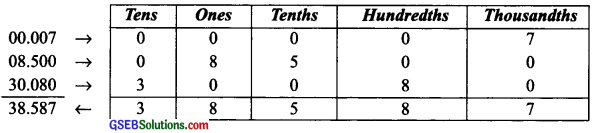Thus, 0.007 + 8.5 + 30.08 = 38.587

(b) 15 + 0.632 + 13.8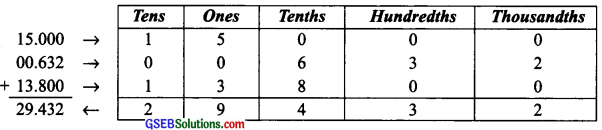Thus, 15 + 0.632 + 13.8 = 29.432

(c) 27.076 + 0.55 + 0.004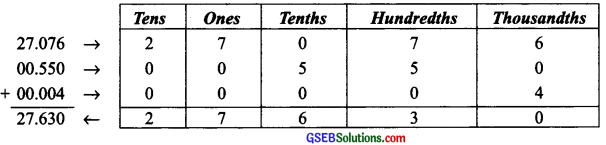Thus, 27.076 + 0.55 + 0.004 = 27.63

(d) 25.65 + 9.005 + 3.7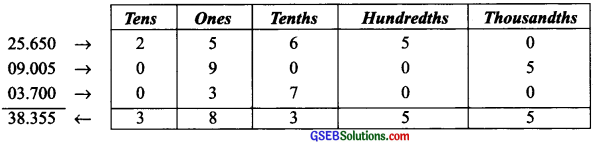Thus, 25.65 + 9.005 + 3.7 = 38.355

(e) 0.75 + 10.425 + 2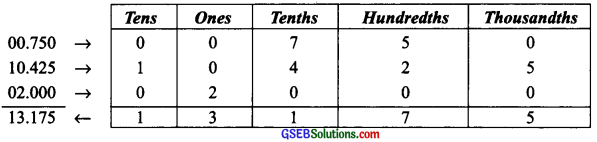Thus, 0.75 + 10.425 + 2 = 13.175

(f) 280.69 + 25.2 + 38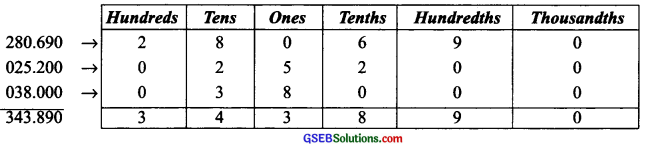Thus, 280.69 + 25.2 + 38 = 343.89Question 2.
Rashid spent? 35.75 for Maths book and? 32.60 for Science book. Find the total amount spent by Rashid.
Solution:
∵ Amount spent
on Maths book = ₹ 35.75
on Science book = ₹ 32.60
∴ Total amount spent = ₹ (35.75 + 32.60) = ₹ 68.35Question 3.
Radhika s mother gave her ₹ 10.50 and her father gave her ₹ 15.80, find the total amount given to Radhika by the parents.
Solution:
The amount is given to Radhika
by her mother = ₹ 10.50
by her father = ₹ 15.80
The total amount is given to her = ₹ 26.30Question 4.
Nasreen bought 3 m 20 cm cloth for her shirt and 2 m 5 cm cloth for her trouser. Find the total length of cloth bought by her.
Solution:
Length of cloth bought:
for her shirt = 3 m 20 cm
for her trouser = 2 m 5 cm
∴ Total length of cloth bought = (3 m 20 cm) + (2 m 5 cm)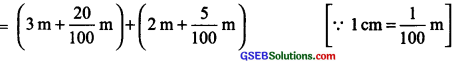= (3 + 0.20) m + (2 + 0.05) m = 3.20 m + 2.05 m = 5.25 mQuestion 5.
Naresh walked 2 km 35 m in the morning and 1 km 7 m in the evening. How much distance did he walk in all?
Solution:
Distance walked by Naresh:
in the morning = 2 km 35 m, in the evening = 1 km 7 m
∴ Total distance walked by Naresh
= (2 km 35 m) + (1 km 7 m)
= (2 km + 35 m) + (1 km + 7 m)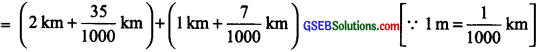= (2 + 0.035) km + (1 + 0.007) km = 3.042 kmQuestion 6.
Sunita traveled 15 km 268 m by bus, 7 km 7 m by car, and 500 m on foot in order to reach her school. How far is her school from her residence?
Solution:
Distance covered:
by bus = 15 km 268 m
by car = 7 km 7 m
on foot = 500 m
∴ Total distance covered = (15 km 268 m) + (7 km 7m) + 500 m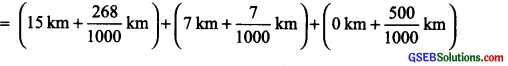= (15 + 0.268) km + (7 + 0.007) km + (0.500) km = 15.268 km + 7.007 km + 0.500 km 15.268
= 22.775 km
Thus, the required distance = 22.775 km.Question 7.
Ravi purchased 5 kg 400 g rice, 2 kg 20 g sugar, and 10 kg 850 g flour. Find the total weight of his purchases.
Solution:
Weight of rice = 5 kg 400 g
Weight of sugar = 2 kg 20 g
Weight of flour = 10 kg 850 g
∴ Total weight = (5 kg 400 g) + (2 kg 20 g) + (10 kg 850 g)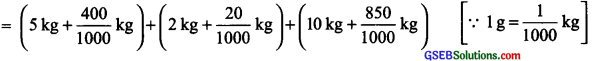= (5 + 0.400) kg + (2 + 0.020) kg + (10 + 0.850) kg
= 5.400 kg + 2.020 kg + 10.850 kg = 18.270 kg
Thus, the total weight of Ravi’s purchases = 18.270 kg.